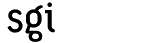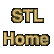# findCategory: algorithms Component type: function

### Prototype

```template<class InputIterator, class EqualityComparable>
InputIterator find(InputIterator first, InputIterator last,
const EqualityComparable& value);
```

### Description

Returns the first iterator i in the range [first, last) such that *i == value. Returns last if no such iterator exists.

### Definition

Defined in the standard header algorithm, and in the nonstandard backward-compatibility header algo.h.

### Requirements on types

• EqualityComparable is a model of EqualityComparable.
• InputIterator is a model of InputIterator.
• Equality is defined between objects of type EqualityComparable and objects of InputIterator's value type.

### Preconditions

• [first, last) is a valid range.

### Complexity

Linear: at most last - first comparisons for equality.

### Example

```list<int> L;
L.push_back(3);
L.push_back(1);
L.push_back(7);

list<int>::iterator result = find(L.begin(), L.end(), 7);
assert(result == L.end() || *result == 7);
```

### Notes